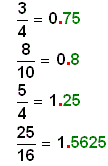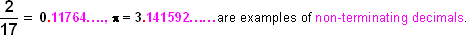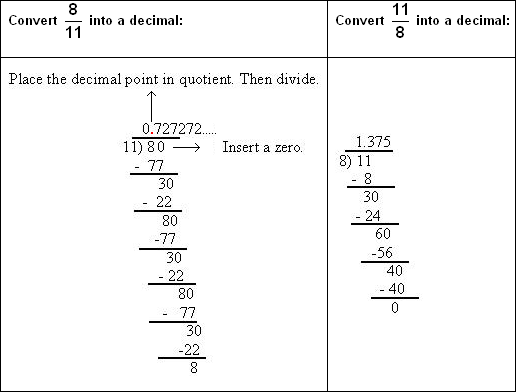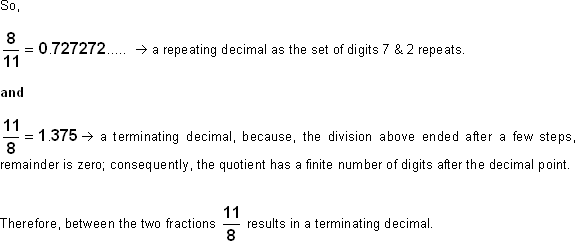Definition Of Terminating Decimal

The word "terminate" means "end".
A decimal that ends is a terminating decimal.

In other words, a terminating decimal doesn't keep going. A terminating decimal will have a finite number of digits after the decimal point.

Examples of Terminating DecimalIn the examples listed above, we have a few fractions expressed as decimals. Notice that these decimals have a FINITE NUMBER OF DIGITS after the decimal point. So, they are terminating decimals.
However, there are situations when the digits after the decimal point DOES NOT END or they go on forever. They are called non-terminating decimals.Solved Example on Terminating Decimal

Solution:

Let's first convert the fractions into decimals by dividing the numerator by the denominator.